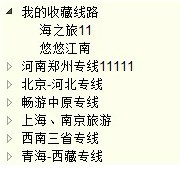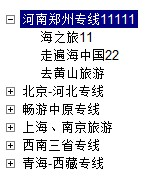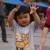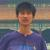## jstree 怎么改变默认的小三角的样式0function(categories,category_id){

var sibling=this._siblingNode(categories, category_id);

var loop=1;

var data='';
if(sibling.length>0){

data+='<ul class="node-list"> \n';

for(var i=0;i<sibling.length;i++){

if(loop==sibling.length){

data+='<li class="end-node"><div>'+sibling[i].name+'</div>'+this.nodeNesting(categories,sibling[i].category_id)+'</li>\n';
}else{
data+='<li class="normal-node"><div>'+sibling[i].name+'</div>'+this.nodeNesting(categories,sibling[i].category_id)+'</li>\n';
}

loop++;

this._loop++;
}
data+='</ul>\n';
}

return data;

}
0